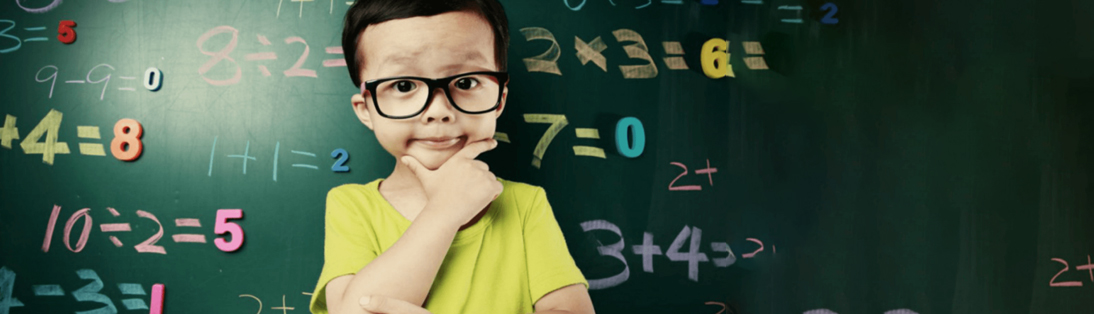• ## Certificate Central# Basics of Vedic Mathematics

Nurture the Genius Within You

The basis of Vedic maths, are the 16 sutras, which attribute a set of qualities to a number or a group of numbers. The ancient Hindu scientists (Rishis) of Bharat in 16 Sutras (Phrases) and 120 words laid down simple steps for solving all mathematical problems in easy to follow 2 or 3 steps. Vedic Mental or one or two line methods can be used effectively for solving divisions, reciprocals, factorisation, HCF, squares and square roots, cubes and cube roots, algebraic equations, multiple simultaneous equations, quadratic equations, cubic equations, biquadratic equations, higher degree equations, differential calculus, Partial fractions, Integrations, Pythagoras Theorem, Apollonius Theoram, Analytical Conics and so on.NUMBER SUTRAS MEANING
1 EKADHIKENA PURVENA One more than the previous one.
2 NIKHILAM NAVATASCARAMAM DASATAH All from 9 and last from 10.
3 URDHVA-TIRYAGBHYAM Criss-cross (Vertically and cross-wise)
4 PARAVARTYA YOJAYET Transpose and adjust.
5 SUNYAM SAMYASAMUCCAYE When the sum is the same, the Sum, i.e it should be equated to zero.
6 SUNYAMANYAT If one is in ratio, the other one is zero.
7 SANKALANA-VYAVAKALANABHYAM By addition and subtraction.
8 PURANAPURANABHYAM By the completion or non-completion.
9 CHALANA KALANABYHAM Difference and similarities.
10 YAVADUNAM Whatever the extent of its deficiency.
11 VYASHTISAMANSTIH Part and whole.
12 SHESANYANKENA CHARAMENA The remainders by the last digit.
13 SOPAANTYADVAYAMANTYAM The ultimate and twice the penultimate.
14 EKANYUNENA PURVENA By one less than the previous.
15 GUNITA SAMUCHAYA The product of the sum is equal to the sum of the product.
16 GUNAKASAMUCHYA The factor of the sum is equal to the sum of the factors.